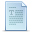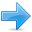# Polymorphism

Before getting into this section, it is recommended that you have a proper understanding of pointers and class inheritance. If any of the following statements seem strange to you, you should review the indicated sections:

Statement:Explained in:
int a::b(int c) { }Classes
a->bData Structures
class a: public b { };Friendship and inheritance

### Pointers to base class

One of the key features of derived classes is that a pointer to a derived class is type-compatible with a pointer to its base class. Polymorphism is the art of taking advantage of this simple but powerful and versatile feature, that brings Object Oriented Methodologies to its full potential.

We are going to start by rewriting our program about the rectangle and the triangle of the previous section taking into consideration this pointer compatibility property:

 ``````// pointers to base class #include using namespace std; class CPolygon { protected: int width, height; public: void set_values (int a, int b) { width=a; height=b; } }; class CRectangle: public CPolygon { public: int area () { return (width * height); } }; class CTriangle: public CPolygon { public: int area () { return (width * height / 2); } }; int main () { CRectangle rect; CTriangle trgl; CPolygon * ppoly1 = ▭ CPolygon * ppoly2 = &trgl; ppoly1->set_values (4,5); ppoly2->set_values (4,5); cout << rect.area() << endl; cout << trgl.area() << endl; return 0; }`````` ```20 10```

In function main, we create two pointers that point to objects of class CPolygon (ppoly1 and ppoly2). Then we assign references to rect and trgl to these pointers, and because both are objects of classes derived from CPolygon, both are valid assignment operations.

The only limitation in using *ppoly1 and *ppoly2 instead of rect and trgl is that both *ppoly1 and *ppoly2 are of type CPolygon* and therefore we can only use these pointers to refer to the members that CRectangle and CTriangle inherit from CPolygon. For that reason when we call the area() members at the end of the program we have had to use directly the objects rect and trgl instead of the pointers *ppoly1 and *ppoly2.

In order to use area() with the pointers to class CPolygon, this member should also have been declared in the class CPolygon, and not only in its derived classes, but the problem is that CRectangle and CTriangle implement different versions of area, therefore we cannot implement it in the base class. This is when virtual members become handy:

### Virtual members

A member of a class that can be redefined in its derived classes is known as a virtual member. In order to declare a member of a class as virtual, we must precede its declaration with the keyword virtual:

 ``````// virtual members #include using namespace std; class CPolygon { protected: int width, height; public: void set_values (int a, int b) { width=a; height=b; } virtual int area () { return (0); } }; class CRectangle: public CPolygon { public: int area () { return (width * height); } }; class CTriangle: public CPolygon { public: int area () { return (width * height / 2); } }; int main () { CRectangle rect; CTriangle trgl; CPolygon poly; CPolygon * ppoly1 = ▭ CPolygon * ppoly2 = &trgl; CPolygon * ppoly3 = &poly; ppoly1->set_values (4,5); ppoly2->set_values (4,5); ppoly3->set_values (4,5); cout << ppoly1->area() << endl; cout << ppoly2->area() << endl; cout << ppoly3->area() << endl; return 0; }`````` ```20 10 0```

Now the three classes (CPolygon, CRectangle and CTriangle) have all the same members: width, height, set_values() and area().

The member function area() has been declared as virtual in the base class because it is later redefined in each derived class. You can verify if you want that if you remove this virtual keyword from the declaration of area() within CPolygon, and then you run the program the result will be 0 for the three polygons instead of 20, 10 and 0. That is because instead of calling the corresponding area() function for each object (CRectangle::area(), CTriangle::area() and CPolygon::area(), respectively), CPolygon::area() will be called in all cases since the calls are via a pointer whose type is CPolygon*.

Therefore, what the virtual keyword does is to allow a member of a derived class with the same name as one in the base class to be appropriately called from a pointer, and more precisely when the type of the pointer is a pointer to the base class but is pointing to an object of the derived class, as in the above example.

A class that declares or inherits a virtual function is called a polymorphic class.

Note that despite of its virtuality, we have also been able to declare an object of type CPolygon and to call its own area() function, which always returns 0.

### Abstract base classes

Abstract base classes are something very similar to our CPolygon class of our previous example. The only difference is that in our previous example we have defined a valid area() function with a minimal functionality for objects that were of class CPolygon (like the object poly), whereas in an abstract base classes we could leave that area() member function without implementation at all. This is done by appending =0 (equal to zero) to the function declaration.

An abstract base CPolygon class could look like this:

 ``123456789`` ``````// abstract class CPolygon class CPolygon { protected: int width, height; public: void set_values (int a, int b) { width=a; height=b; } virtual int area () =0; };``````

Notice how we appended =0 to virtual int area () instead of specifying an implementation for the function. This type of function is called a pure virtual function, and all classes that contain at least one pure virtual function are abstract base classes.

The main difference between an abstract base class and a regular polymorphic class is that because in abstract base classes at least one of its members lacks implementation we cannot create instances (objects) of it.

But a class that cannot instantiate objects is not totally useless. We can create pointers to it and take advantage of all its polymorphic abilities. Therefore a declaration like:

 ``1`` ``CPolygon poly;``

would not be valid for the abstract base class we have just declared, because tries to instantiate an object. Nevertheless, the following pointers:

 ``12`` ``````CPolygon * ppoly1; CPolygon * ppoly2;``````

would be perfectly valid.

This is so for as long as CPolygon includes a pure virtual function and therefore it's an abstract base class. However, pointers to this abstract base class can be used to point to objects of derived classes.

Here you have the complete example:

 ``````// abstract base class #include using namespace std; class CPolygon { protected: int width, height; public: void set_values (int a, int b) { width=a; height=b; } virtual int area (void) =0; }; class CRectangle: public CPolygon { public: int area (void) { return (width * height); } }; class CTriangle: public CPolygon { public: int area (void) { return (width * height / 2); } }; int main () { CRectangle rect; CTriangle trgl; CPolygon * ppoly1 = ▭ CPolygon * ppoly2 = &trgl; ppoly1->set_values (4,5); ppoly2->set_values (4,5); cout << ppoly1->area() << endl; cout << ppoly2->area() << endl; return 0; }`````` ```20 10```

If you review the program you will notice that we refer to objects of different but related classes using a unique type of pointer (CPolygon*). This can be tremendously useful. For example, now we can create a function member of the abstract base class CPolygon that is able to print on screen the result of the area() function even though CPolygon itself has no implementation for this function:

 ``````// pure virtual members can be called // from the abstract base class #include using namespace std; class CPolygon { protected: int width, height; public: void set_values (int a, int b) { width=a; height=b; } virtual int area (void) =0; void printarea (void) { cout << this->area() << endl; } }; class CRectangle: public CPolygon { public: int area (void) { return (width * height); } }; class CTriangle: public CPolygon { public: int area (void) { return (width * height / 2); } }; int main () { CRectangle rect; CTriangle trgl; CPolygon * ppoly1 = ▭ CPolygon * ppoly2 = &trgl; ppoly1->set_values (4,5); ppoly2->set_values (4,5); ppoly1->printarea(); ppoly2->printarea(); return 0; }`````` ```20 10```

Virtual members and abstract classes grant C++ the polymorphic characteristics that make object-oriented programming such a useful instrument in big projects. Of course, we have seen very simple uses of these features, but these features can be applied to arrays of objects or dynamically allocated objects.

Let's end with the same example again, but this time with objects that are dynamically allocated:

 ``````// dynamic allocation and polymorphism #include using namespace std; class CPolygon { protected: int width, height; public: void set_values (int a, int b) { width=a; height=b; } virtual int area (void) =0; void printarea (void) { cout << this->area() << endl; } }; class CRectangle: public CPolygon { public: int area (void) { return (width * height); } }; class CTriangle: public CPolygon { public: int area (void) { return (width * height / 2); } }; int main () { CPolygon * ppoly1 = new CRectangle; CPolygon * ppoly2 = new CTriangle; ppoly1->set_values (4,5); ppoly2->set_values (4,5); ppoly1->printarea(); ppoly2->printarea(); delete ppoly1; delete ppoly2; return 0; }`````` ```20 10```

Notice that the ppoly pointers:

 ``12`` ``````CPolygon * ppoly1 = new CRectangle; CPolygon * ppoly2 = new CTriangle;``````

are declared being of type pointer to CPolygon but the objects dynamically allocated have been declared having the derived class type directly.Previous:Friendship and inheritanceIndexNext:Templates